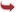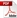International Journal of Scientific and Research Publications

#### IJSRP, Volume 8, Issue 3, March 2018 Edition [ISSN 2250-3153]Pure Bending Analysis of Isotropic Thin Rectangular Plates Using Third-Order Energy Functional
Emmanuel C. Oba, Pius C. Anyadiegwu, Abednego G. T. George, Ethelbert C. Nwadike
Abstract: Studies previously carried out on pure bending analysis of isotropic thin rectangular plate using total potential energy functional have mainly used second order (Ritz energy function) and fourth order (Galerkin and work error energy functional). The objective of this study is pure bending analysis of isotropic thin rectangular CCCC, CSCS, CSSS and SCCC plates. In this paper, third order energy functional is used for analysis. The Rayleigh Ritz energy method of direct variation approach for plate analysis is adopted. The third order energy functional method is derived from first principle by using equations and principles of theory of elasticity. Polynomial series is used to formulate the approximate shape functions for the plate with various boundary conditions. Direct variational calculus was applied on the third-order energy function to obtain the coefficient of deflection which was used to determine the stiffness component for the plates. The coefficients of deflection for CCCC, CSCS, CSSS and SCCC are obtained for various aspect ratios (ranging from 1.0 to 2.0 at the increment of 0.1). The various coefficient of deflection is used to obtain the deflection at the center of the plates. The central deflection of the various plate with aspect ratio (ranging from 1.0 to 2.0 at the increment of 0.1) from this studies were compared with the values of Szilard (2004) and Timoshenko and Krieger (1959). From the comparison, it was observed that the maximum percentage difference recorded for CCCC, CSCS and CSSS plates was 3.58, 4.91 and 1.79 respectively. While for SCCC there was no significant difference. These values of percentage differences are relatively small, which indicates that the method of this present study is considered adequate, satisfactory and reliable for analyzing pure bending isotropic thin rectangular plate.
[VIEW FULL PAPER][DOWNLOAD]

Reference this Research Paper (copy & paste below code):

Emmanuel C. Oba, Pius C. Anyadiegwu, Abednego G. T. George, Ethelbert C. Nwadike (2018); Pure Bending Analysis of Isotropic Thin Rectangular Plates Using Third-Order Energy Functional; Int J Sci Res Publ 8(3) (ISSN: 2250-3153). http://www.ijsrp.org/research-paper-0318.php?rp=P757279